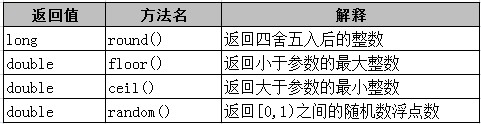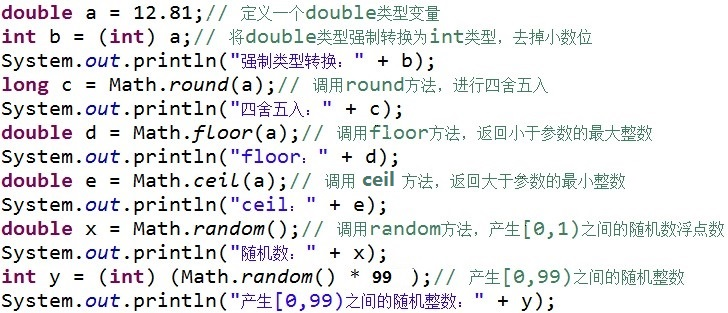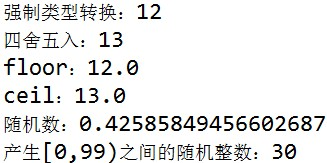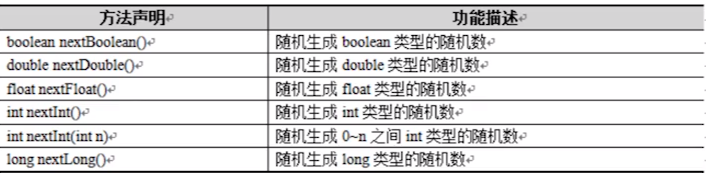• Math类在编程中常被用于计算商业驱动程序、动态显示布局、用于游戏界面/功能的随机活动，以及程序需要的代数和三角函数功能。高级的数学计算需要使用到函数和常量方程。Math类包含了各种方法和常量，用于协助解决...
          大家好。这是我的第一篇博文，其实是我的老师布置的作业。好吧，闲话少说，下面进入正题。我学的是JAVA，所以就来说说JAVA中的Math类吧。Math类在编程中常被用于计算商业驱动程序、动态显示布局、用于游戏界面/功能的随机活动，以及程序需要的代数和三角函数功能。高级的数学计算需要使用到函数和常量方程。Math类包含了各种方法和常量，用于协助解决数学计算。阅读完本章，你将非常轻松自如地使用Math类。
使用Math类不仅仅是进行加减乘除。Math的各种方法和常量可以进行算数和三角计算，以及产生随机数。这些方法和常量与计算器上的按键很相似，比如正弦、正切和 。Math类是一个静态类，因此不必声明一个Math类的对象再使用其方法和常量。不必使用new创建一个类对象注册成为类成员，而只需要在类本身调用方法或属性即可，就像这样：Math. method();或Math.CONSTANT;。Math类同时也包括不会改变的静态常量，比如PI（3.141592653589793）和E（2.71828182845905）。可以在Flash的帮助文档中查询"Math
class"查看。
Math类基本方法

1.       Math.abs(int a)：返回值的绝对值。参数可以的类型int 、float、double、long
2.       Math.acos(double a)：返回角的反余弦。
3.       Math.asin(double a)：返回角的反正弦。
4.       Math.atan(double a)：返回角的反正切。
5.       Math.atan2(double a)：将矩形坐标（x,y）转换成极坐标（r,theta）。
6.       Math.cbrt(double a)：返回double 值的立方根。
7.       Math.ceil(double a)：返回最小的（最接近负无穷大）double值，该值大于或等于参数，并且等于某个整数。
8.       Math.cos(double a)：返回角的三角余弦。
9.       Math.cosh(double x)：返回double 值的双曲线余弦。
10.   Math.exp(double a)：返回欧拉数e 的double 次幂的值。
11.   Math.floor(double a)：返回最大的（最接近正无穷大）double 值，该值小于或等于参数，并且等于某个整数。
12.   Math.max(int a,int b)：返回两个值中较大的一个值。参数包含的类型 int、float、long、double。
13.   Math.min(int a,int b)：返回两个值中较小的一个值。参数包含的类型 int、float、long、double。
14.   Math.random()：返回带正号的double值。
15.   Math.round(double a)：返回最接近参数的long。
16.   Math.round(float a)：返回最接近参数的int。
Math类的误区
性能测试：
var num:Number = Math.PI;
var length:int = 10000000;
var time:int = getTimer();
for(var i:int = 0; i < length; i ++){
Math.floor(num);
}
trace(getTimer() - time);
// 结果：1865
var num:Number = Math.PI;
var length:int = 10000000;
var time:int = getTimer();
for(var i:int = 0; i < length; i ++){
int(num);
}
trace(getTimer() - time);
// 结果：69
结果很明显，int比floor快，可能大家就要说了，那Adobe傻X写个这样接口干什么？其实这就是我想说的对于floor的使用误区。
仔细看官方解释，其实floor不是一个真正我们所理解的取整函数，他是去找最接近自己，且比自己小或者等于的整数，那这是什么意思呢？
var num:Number = - Math.PI;
trace(int(num));
trace(Math.floor(num));
// 结果1：-3
// 结果2：-4
这个例子很明确表示，当目标数字是负数时，int和floor所得出的结果不一样。

int()的官方解释
将给定数字值转换成整数值。 从小数点处截断十进制值。

相信看到这，你已经很明白了。
但是实际上，如果你这样去使用int()，跟floor输出的结果相同：
var num:Number = - Math.PI;
var length:int = 10000000;
var time:int = getTimer();
for(var i:int = 0; i < length; i ++){
if(num < 0){
int(num) - 1;
}else{
int(num);
}
}
trace(getTimer() - time);
// 结果：132

2、Math.pow()
对于这个方法，我也不知道说什么好了，先看性能测试吧：
var a:int = 3;
var b:int = 4;
var c:int = 5;
var length:int = 10000000;
var time:int = getTimer();
for(var i:int = 0; i < length; i ++){
c * c == a * a + b * b;
}
trace(getTimer() - time);
// 结果：95
var a:int = 3;
var b:int = 4;
var c:int = 5;
var length:int = 10000000;
var time:int = getTimer();
for(var i:int = 0; i < length; i ++){
Math.pow(c,2) == Math.pow(a,2) + Math.pow(b,2);
}
trace(getTimer() - time);
// 结果：7999
勾三股四弦五大家应该比较多，但是用"*"乘法运算和pow的性能比较那是非常明显啊。
难道大家又想说Adobe傻逼了？这儿我为它平反吧。
var num:Number = Math.PI;
var length:int = 10000000;
var time:int = getTimer();
for(var i:int = 0; i < length; i ++){
Math.pow(num,10000);
}
trace(getTimer() - time);
// 结果：6682
先不说"*"乘法运算比pow快，就上面这段你能把它换算成使用"*"乘法运算吗？
而且pow其实是可以这样用的：（数学学得好都知道开方其实是可以转换成乘方来算的）
trace(Math.pow(27,1/3));
trace(Math.pow(256,1/4));
trace(Math.pow(3125,1/5));
而开方函数Adobe只提供了sqrt一个开平方根的接口（经测试Math.sqrt(9)比Math.pow(9,1/2)快，但开立方等就得靠pow了）。

3、Math.round()
这个方法跟floor一样的，先看性能测试：
var num:int = Math.PI;
var length:int = 10000000;
var time:int = getTimer();
for(var i:int = 0; i < length; i ++){
Math.round(num);
}
trace(getTimer() - time);
// 结果：1931
var num:int = Math.PI;
var length:int = 10000000;
var time:int = getTimer();
for(var i:int = 0; i < length; i ++){
int(num + 0.5)
}
trace(getTimer() - time);
// 结果：68
四舍五入其实加个0.5在取整，这样也是可以的，只不过用这个算法，存在跟第一个同样的问题，当目标数值为负时，两种方式结果不一样，需要加个判断，目标数值为负就把结果。     就到这吧，其实我也是上网找的。


展开全文• Math 的方法都被定义为 static 形式，通过 Math 可以在主函数直接调用。 不需要导包:java.lang下的直接不需要导包。 random方法: Math.random() 随机生成[0,1)小数 公式: [0,max) 随机整数 ...
Java Math 类
Java 的 Math 包含了用于执行基本数学运算的属性和方法，如初等指数、对数、平方根和三角函数。
Math 的方法都被定义为 static 形式，通过 Math 类可以在主函数中直接调用。
不需要导包:java.lang下的直接类不需要导包。
random方法:
Math.random()           随机生成[0,1)小数
公式:
[0,max) 随机整数  (int)(Math.random()max)
[0,max] 随机整数  (int)(Math.random()(max+1))
[min,max) 随机整数  (int)(Math.random()(max-min)+min)
[min,max] 随机整数  (int)(Math.random()(max-min+1)+min)
​	Math.round(数据) 四舍五入
​	Math.abs(数据); 绝对值
​	Math.sqrt(数据); 平方根  double类型的值
实例1:
public class Demo{
/**主方法*/
public static void main(String[] args){
double num1=(int)Math.random();
System.out.println(num1);

//----------------------------------------------------------------
//[0,5) 随机整数  最终的结果取整强转
int num2=(int)(Math.random()*5); //[0,1) *5  ->[0,5)
//----------------------------------------------------------------
//[0,5]
num2= (int)(Math.random()*(5+1));
System.out.println(num2);
//----------------------------------------------------------------
//[3,7)
num2= (int)(Math.random()*(7-3)+3);  //[0,4)+3 -->[3,7)
System.out.println(num2);
//----------------------------------------------------------------
System.out.println(Math.round(3.49));//四舍五入
System.out.println(Math.abs(-33.3));//绝对值
System.out.println(Math.sqrt(2));//平方根  为double类型的值
//----------------------------------------------------------------
/*
例题:
随机生成一个小写字母,判断这个字符是否为元音 a e i o u
思路:
//1.随机产生一个字母 小写
//2.判断  if..else   switch
*/

//1.随机产生一个字母 小写  [97,122]
int num=(int)(Math.random()*(122-97+1)+97);
char ch=(char)num;

//2.判断 利用if..else  或者  switch
if(ch=='a' || ch=='e' || ch=='i' || ch=='o' || ch=='u'){
System.out.println(ch+"是元音");
}else {
System.out.println(ch+"是辅音");
}
//或
switch(ch){
case 'a':
case 'e':
case 'i':
case 'o':
case 'u':
System.out.println(ch+"是元音");
break;
default:
System.out.println(ch+"是辅音");
break;
}
}
}

实例2:
public class MathDemo01 {
public static void main(String[] args) {
//static double ceil(double a)   向上取整
System.out.println(Math.ceil(-3.9));
//floor(double a) 向下取整
System.out.println(Math.floor(-3.3));

//max(参数1,参数2)
//min(参数1,参数2)
long l1=123;
byte b1=101;
System.out.println(Math.max(l1,1.1));
System.out.println(Math.min(l1,1.1));

//static long round(double a) 四舍五入,只作用到小数点后一位
System.out.println(Math.round(1.59));
}
}



展开全文• Math 类位于 java.lang 包中，包含用于执行基本数学运算的方法， Math 类的所有方法都是静态方法，所以使用该类中的方法时，可以直接使用类名.方法名，如： Math.round();常用的方法：通过案例我们来认识一下他们的...
Math 类位于 java.lang 包中，包含用于执行基本数学运算的方法， Math 类的所有方法都是静态方法，所以使用该类中的方法时，可以直接使用类名.方法名，如： Math.round();常用的方法：通过案例我们来认识一下他们的使用吧！！运行结果：PS： Math 类还提供了许多其他方法，各位小伙伴们可以注意关注 wiki ，查阅更多信息示例：定义一个包含 10 个元素的整型数组，通过随机产生 10 以内的随机数，给数组中的每个元素赋值，并输出结果public class HelloWorld {public static void main(String[] args) {// 定义一个整型数组，长度为10int[] nums = new int;//通过循环给数组赋值for (int i = 0; i < nums.length; i++) {// 产生10以内的随机数int x = (int)(Math.random()*10);nums[i] = x;// 为元素赋值}// 使用foreach循环输出数组中的元素for ( int num : nums) {System.out.print(num + " ");}}}输出：6 0 6 3 2 7 7 2 7 6
展开全文• Math类中有两个静态常量PI和E分别代表数学常量π和e。 Random类 可以在指定的取值范围内随机产生数字 Random的构造方法 第一种 Random() 用于创建一个伪随机数生成器 该方法创建时使用的种子是随机的，每个...
Java API学习记录——Math类和Random类

Math类

Math类时数学操作类，提供了一系列用于数学运算的静态方法，包括求绝对值、三角函数等。Math类中有两个静态常量PI和E分别代表数学常量π和e。

Random类

可以在指定的取值范围内随机产生数字

Random的构造方法

第一种

Random()

用于创建一个伪随机数生成器

该方法创建时使用的种子是随机的，每个对象产生的随机数不同

第二种

Random(long seed)

构造方法，使用一个long型的seed种子创建伪随机数生成器

该方法传入了一个固定的种子，每个实例化的Random对象产生相同序列的随机数

常用方法展开全文java random
• Random类中实现的随机算法是伪随机，也就是有规则的随机。在进行随机时，随机算法的起源数字称为种子数(seed)，在种子数的基础上进行一定的变换，从而产生需要的随机数字。相同种子数的Random对象，相同次数生成的...java Random类
• Math类中存在一个random（）方法，用于产生随机数字，这个方法默认生成大于等于 0.0 且小于 1.0 的double型随机数；即 0 <= Math.random() < 1.0 ，虽然Math.random() 方法只可以产生0 ~ 1之间的double型...java
• Math类中存在一种random（）方法，用于产生随机的数字和字符 ①随机数字的产生 random（）语法 (int)(Math.random()*n): 返回大于等于0且小于n的随机数 m+(int)(Math.random()*n): 返回大于等于m且小于m+n(不包括m+n...java random
• Math是一个工具类，主要用于完成复杂的数学运算，Math类中的所有方法都是静态方法，可以直接通过类名开调用他们。 在JDK的Java.util包中，有一个Random类，它可以在指定的取值范围内随机产生数字，在Random类中提供...
• Random类比Math类的random()方法提供了更多的方式来生成各种伪随机数，可以生成浮点类型的伪随机数，也可以生成整数类型的伪随机数，还可以指定生成随机数的范围。构造函数：public Random() //...
• Math类中存在一个random()方法，用于产生随机数字，这个方法默认生成大于等于0.0小于1.0的double型随机数，即0&lt;=Math.random()&lt;1.0，虽然Math.random()方法只可以产生0~1之间的double型数字，其实...
• 0.0 在Math类中存在一个random()方法，用于产生随机数字，这个方法生成在0.0<=生成数<1.0 0.1那么它默认的生成的数显然在有些时候是满足不了我们的，我们来看看它的升级版 类型 返回值 (int)(Math....
• 数字处理类1、前言2、Math类2.1、三角函数方法2.2、指数函数方法2.3、取整函数方法2.4、最大值、最小值...Java提供了处理相关问题的类，如用于格式化数字的DecimalFormat类，数学计算相关的Math类，随机数相关的Rand
• Math类的方法都被定义为静态形式，通过Math类可以在主函数直接调用。 Random类 Random()：用于创建一个伪随机数产生器。 Random random=new Random(); System.out.printf(random.nextInt(100)+"");//随机...
• Math类中提供了一个random()的方法,用于随机一个从0.0~1.0之间的浮点数，当实际需求有个性化的要求时，只能通过计算获取其他类型的随机数，实际操作相对比较麻烦；因此，Java中还提供了另一个专门用于生成各种需求的...java
• 接上一篇使用 Math 类操作数据 Math 类位于 java.lang 包中，包含用于执行基本数学运算的方法， Math 类的所有方法都是静态方法，所以使用该类中的方法时，可以直接使用类名.方法名，如： Math.round(); 常用的方法...java四舍五入
• 课程部分 主要讲 Nueral Network ...2 under sample the majority class(欠采样，通常用于不平衡数据集，在fit 时随机删除一部分imbalance data的多数) 3using model metrics (accuracy, percision, recall, AUC a
• 今天我们谈一谈JAVA中的随机数随机数 ——Math.random()0.0 在Math类中存在一个random()方法，用于产生随机数字，这个方法生成在0.0<=生成数<1.00.1那么它默认的生成的数显然在有些时候是满足不了我们的，我们...
• 展开全部过程如图所2113示：获取1~100以内的随机数有5261两种方法：方法1：可以4102通过Math类中的random方法获取随机数，再乘以100加16531，然后转换为int类型即可。方法2：可以通过Random类中的nextInt方法获取...
• Math是一个工具类，主要用于完成复杂的数学运算，Math类中的所有方法都是静态方法，可以直接通过类名开调用他们。 在JDK的Java.util包中，有一个Random类，它可以在指定的取值范围内随机产生数字，在Random类中提供...java
• 一、随机数 在实际开发中产生随机数的使用普遍存在，所以在程序中进行产生随机数操作很重要。... 1.1 Math.random() 方法 在 Math 类中存在一个 random 方法，用于产生随机数字，这个方法默认生成大...Java基础
• 1、random()方法用于产生随机数字，默认产生0~1之间的double型数字。 public class test { public static int GetRandomNum(double num1, double num2) { // 产生num1和num2之间的随机数 int s = (int) num1 + ...
• 在Java中主要提供了两种方式产生...在Math类中存在一个random()方法，用于产生随机数字，这个方法默认生成大于等于0.0小于1.0的double型随机数，通过以下示例来说明这个方法： public class MathRandom { /*...Java
• 用于生成伪随机数的流。 该使用48位种子，其使用线性同余公式进行修改 许多应用程序会发现方法Math.random()使用起来更简单。 java.util.Random的java.util.Random是线程安全的。 但是，跨线程的同时使用java....java random random原理
• Java提供了处理相关问题的类，包括DecimalFormat类(用于格式化数字)、Math类（为各种数学计数提供了工具方法）、Random类(为Java处理随机数问题提供了各种方法)、BigInteger类与BigDecimal类(为所有大数字的处理提供...Java从入门到精通
• 注意：必须将Math.random()用于此分配的所有随机数。 要求 代表单个细菌或某些其他单个生物的。 该班将有以下成员： 3个整数，它们保留细菌位置的x和y坐标以及细菌的颜色。 初始化3个整数的构造函数 void ...JavaScript
• 1.Math.random()方法：在Math类中存在一个random()方法，用于产生随机数字，这个方法默认生成大于等于0.0小雨1.0的double型随机数,即0 （1）(int)(Marh.random()*n) 返回大于等于0小于n之间的随机数 （2）m+( int )...
• ## Java中数字格式化

千次阅读 2018-08-03 09:00:41
为了应对以上问题，Java提供了处理相关问题的类，包括DecimalFormat类（用于格式化数字）、Math类（为各种数学计算提供了工具方法）、Random类（为Java处理随机数问题提供了分钟方法）、BigInteger类与BigDecimal类...
• [超级链接：Java并发学习系列-绪论]...ThreadLocalRandom是Java提供的用于并发产生伪随机数的工具，出现于JDK1.7版本。 ThreadLocalRandom可以看做Math.random()的并发升级。 ThreadLocalRandom不是直接用ne...Random seed
• Math类 Math 类包含用于执行基本数学运算的方法（数学类） Math 类没有构造方法，全是静态方法，一般在开发很少使用到。 常规的一些方法 产生随机数：random() 【大于等于 0.0 且小于 1.0 的伪随机 double 值】 ...